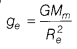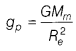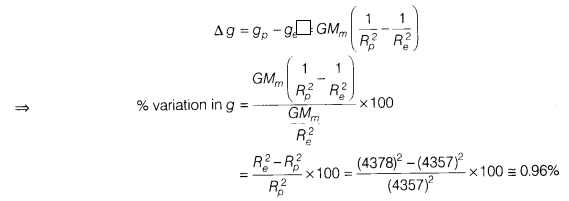# Calculate the percentage change in the weight when it is taken from equator to the poles

If the radius of the Moon at the poles is 4357 km and the radius at the equator is 4378 km, then calculate the percentage change in the weight when it is taken from equator to the poles.

Let acceleration due to gravity at poles,and acceleration due to gravity at poles,The variation of acceleration due to gravity,∴ % variation in the weight of a body = % change in g = 0.96%